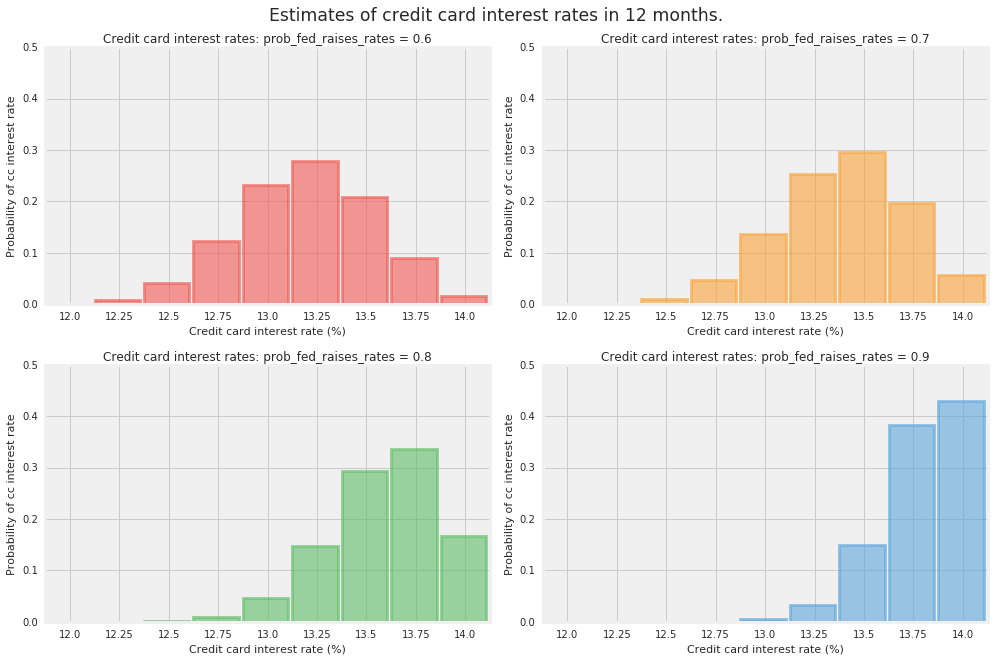# Credit Card Interest

Let’s imagine that you would like to estimate the interest rate on your credit card one year from now. Suppose the current prime rate is 2% and your credit card company charges you 10% plus prime. Given the strength of the current economy, you believe that the Federal Reserve is more likely to raise interest rates than not. The Fed will meet eight times in the next twelve months and will either raise the federal funds rate by 0.25% or leave it at the previous level.

We use the binomial distribution to model your credit card’s interest rate at the end of the twelve-month period. Specifically, we’ll use the TensorFlow Probability Binomial distribution class with the following parameters: total_count = 8 (number of trials or meetings), probs = {.6, .7, .8, .9}, for our range of estimates about the probability of the Fed raising the federal funds rate by 0.25% at each meeting.

### Compute Probabilities

Compute the probabilities of possible credit card interest rates in 12 months.

# First we encode our assumptions.
num_times_fed_meets_per_year = 8.
possible_fed_increases = tf.range(
start=0.,
limit=num_times_fed_meets_per_year + 1)
possible_cc_interest_rates = 2. + 10. + 0.25 * possible_fed_increases
prob_fed_raises_rates = tf.constant([0.6, 0.7, 0.8, 0.9])  # Wild guesses.

# Now we use TFP to compute probabilities in a vectorized manner.
# Pad a dim so we broadcast fed probs against CC interest rates.
prob_fed_raises_rates = prob_fed_raises_rates[..., tf.newaxis]
prob_cc_interest_rate = tfd.Binomial(
total_count=num_times_fed_meets_per_year,
probs=prob_fed_raises_rates).prob(possible_fed_increases)


### Execute TF Code

# Convert from TF to numpy.
[
possible_cc_interest_rates_,
prob_cc_interest_rate_,
prob_fed_raises_rates_,
] = evaluate([
possible_cc_interest_rates,
prob_cc_interest_rate,
prob_fed_raises_rates,
])


### Visualize Results

plt.figure(figsize=(14, 9))
for i, pf in enumerate(prob_fed_raises_rates_):
plt.subplot(2, 2, i+1)
plt.bar(possible_cc_interest_rates_,
prob_cc_interest_rate_[i],
color=TFColor[i],
width=0.23,
label="$p = {:.1f}$".format(pf),
alpha=0.6,
edgecolor=TFColor[i],
lw="3")
plt.xticks(possible_cc_interest_rates_ + 0.125, possible_cc_interest_rates_)
plt.xlim(12, 14.25)
plt.ylim(0, 0.5)
plt.ylabel("Probability of cc interest rate")
plt.xlabel("Credit card interest rate (%)")
plt.title("Credit card interest rates: "
"prob_fed_raises_rates = {:.1f}".format(pf));
plt.suptitle("Estimates of credit card interest rates in 12 months.",
fontsize="x-large",
y=1.02)
plt.tight_layout()[{ "type": "thumb-down", "id": "missingTheInformationINeed", "label":"Missing the information I need" },{ "type": "thumb-down", "id": "tooComplicatedTooManySteps", "label":"Too complicated / too many steps" },{ "type": "thumb-down", "id": "outOfDate", "label":"Out of date" },{ "type": "thumb-down", "id": "samplesCodeIssue", "label":"Samples / code issue" },{ "type": "thumb-down", "id": "otherDown", "label":"Other" }]
[{ "type": "thumb-up", "id": "easyToUnderstand", "label":"Easy to understand" },{ "type": "thumb-up", "id": "solvedMyProblem", "label":"Solved my problem" },{ "type": "thumb-up", "id": "otherUp", "label":"Other" }]